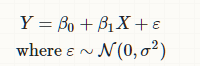# Question on math notation

I found this in a cross validated forum and trying to understand the math notation here.
A standard linear model (e.g., a simple regression model) can be thought of as having two ‘parts’. These are called the structural component and the random component. For example:
Y=β0+β1X+εwhere ε∼N(0,σ2)
Y=β0+β1X+εwhere ε∼N(0,σ2)
The first two terms (that is, β0+β1Xβ0+β1X) constitute the structural component, and the εε (which indicates a normally distributed error term) is the random component.I am not sure what the notation represented by the big “N” is called. I looked in rapidtables but did not see this notation there.

Big ‘N’ is for normal distribution. ε∼N(0,σ2) means ε will have normal distribution with mean ‘0’ and variance ‘σ2’

1 Like

Thank You @Manoj